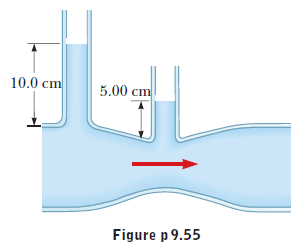# Problem: The inside diameters of the larger portions of the horizontal pipe depicted in the figure are 2.50 cm. Water flows to the right at a rate of 1.80 x 10-4 m3/s. Determine the inside diameter of the constriction.

###### FREE Expert Solution
97% (433 ratings)
###### Problem Details

The inside diameters of the larger portions of the horizontal pipe depicted in the figure are 2.50 cm. Water flows to the right at a rate of 1.80 x 10-4 m3/s. Determine the inside diameter of the constriction.Frequently Asked Questions

What scientific concept do you need to know in order to solve this problem?

Our tutors have indicated that to solve this problem you will need to apply the Bernoulli's Equation concept. If you need more Bernoulli's Equation practice, you can also practice Bernoulli's Equation practice problems.

How long does this problem take to solve?

Our expert Physics tutor, Julia took 8 minutes and 22 seconds to solve this problem. You can follow their steps in the video explanation above.

What professor is this problem relevant for?

Based on our data, we think this problem is relevant for Professor Warncke's class at EMORY.

What textbook is this problem found in?

Our data indicates that this problem or a close variation was asked in College Physics - Serway 10th Edition. You can also practice College Physics - Serway 10th Edition practice problems.